Your browser doesn't support the features required by impress.js, so you are presented with a simplified version of this presentation.

For the best experience please use the latest Chrome, Safari or Firefox browser.

# Normalization of Affymetrix DNA chip

## D. Puthier

Inserm U1090/TAGC

Press F11 for full screen

# Affymetrix DNA Chip

## Technology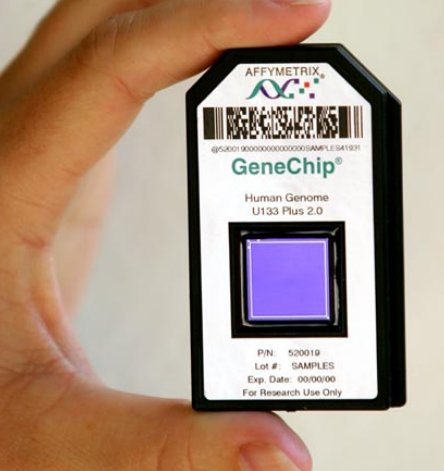• In situ synthesis of oligonucleotides.
• Features.
• Cells: 24µm x 24µm
• ~ 1e7 oligos per cell
• ~ 4e5-1.5e6 probes per chip

# Affymetrix DNA Chip

## Probes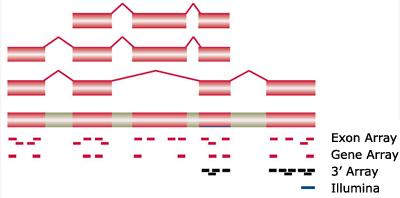• Short oligonucleotides (25 mers)
• 3' arrays (3' IVT Expression Analysis)

• PM and MM
• 10-20 probes/gene

• Exon/Gene arrays (Whole-Transcript Expression Analysis)
• No more MM
• ~4 probes per exon, ~40 per gene (Exon Arrays)
• ~26 probes per gene (Gene arrays)

# Affymetrix DNA Chip

## Mismatch and perfect match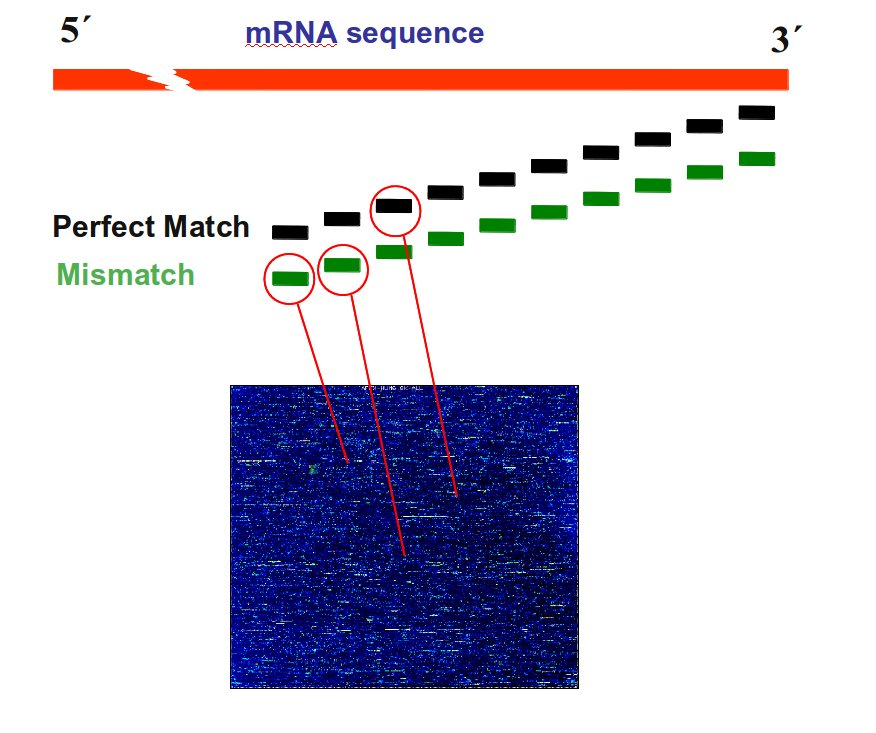• Perfect Match (PM): specific probe
• Mismatch (MM): degenerated probe
• ProbePair: a pair of PM and MM
• ProbeSet: a set of x PM and MM targeting a gene (e.g; U12140_at)# Normalization: main sources of artefact

• RNA quantity / quality
• Labelling efficiency
• Scanner parameters
• Local background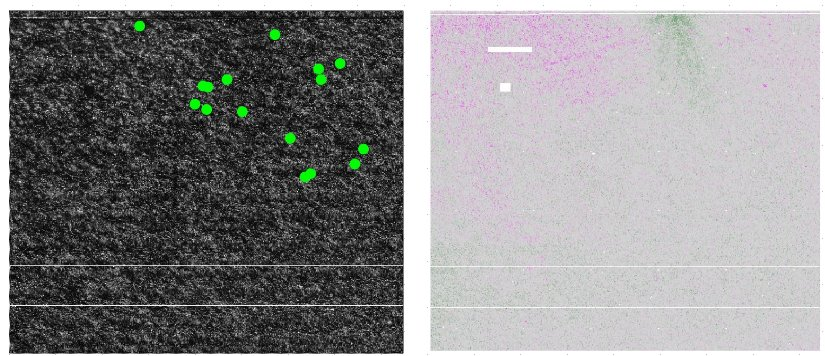# Normalizing columns of a numeric matrix

## Standardization (set mean to 0 and standard deviation to 1 in each column)

• centering: substracting the mean from each value
• scaling: dividing the centered value by the standard deviation
$$z = (x - m_{est}) / s_{est}$$

where
• $$\ z$$ is the standardized value (the z-score)
• $$\ m_{est}$$ is a sample-based estimate of the population mean
• $$\ s_{est}$$ is a sample-based estimate of the population standard deviation

# Normalizing columns of a numeric matrix

## Robust standardization (set median to 0 and median absolute deviation to 1 in each column)

• centering: substracting the median from each value
• scaling: dividing the centered value by the median absolute deviation
$$z = (x - \tilde{m}_{est}) / \tilde{s}_{est}$$

where
• $$\ z$$ is the standardized value
• $$\ \tilde{m}_{est}$$ is a sample-based estimate of the population median
• $$\ \tilde{s}_{est}$$ is a sample-based estimate of the population mad

# Normalizing columns of a numeric matrix

## Quantile normalization

• Force columns to contain the same set of values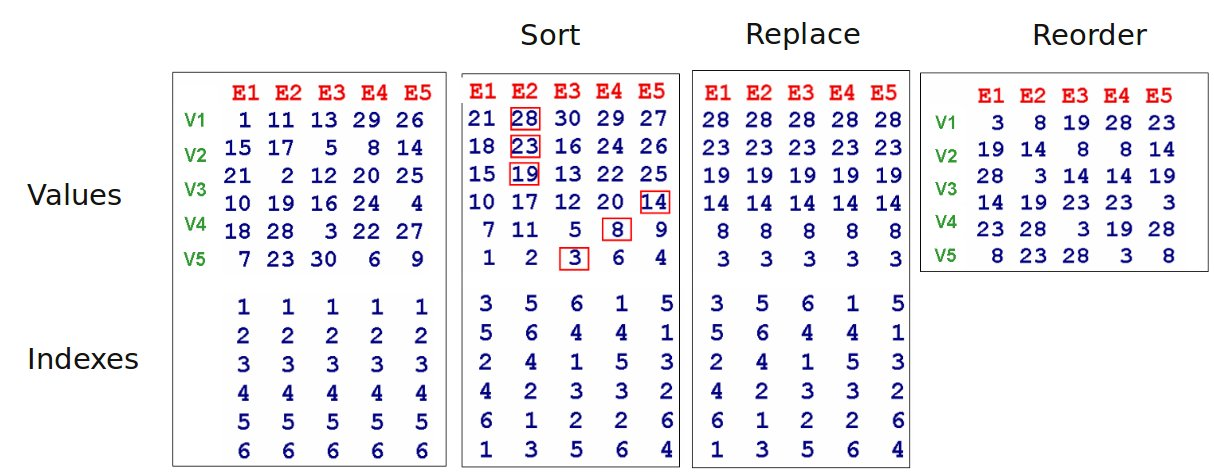# The RMA algorithm

## Robust Multichip Average

The most popular algorithm for Affymetrix data normalization

• MM values are excluded from analysis
• Global background estimation and substraction
• Quantile normalization (probe level)
• Median Polish (summarization)

# The RMA algorithm

## Median polish algorithm

Motivations

• Probes may display higher or lower affinity for targets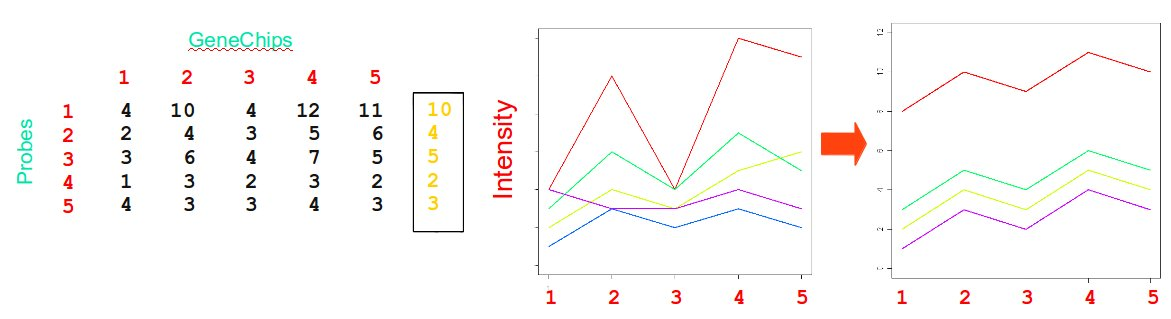# The RMA algorithm

## Median polish algorithm

Procedure

• Compute a residual matrix by iteratively
• Substract the corresponding median from each row
• Substract the corresponding median from each column
• Stop if row medians and column medians are equal to zero
• Subtract the residual matrix from the initial matrix
• Compute the mean of each column to get summarized expression values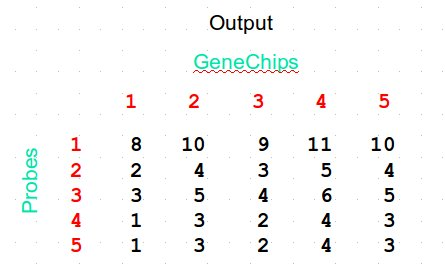• GCRMA
• PLIER
• RefRMA
• ...